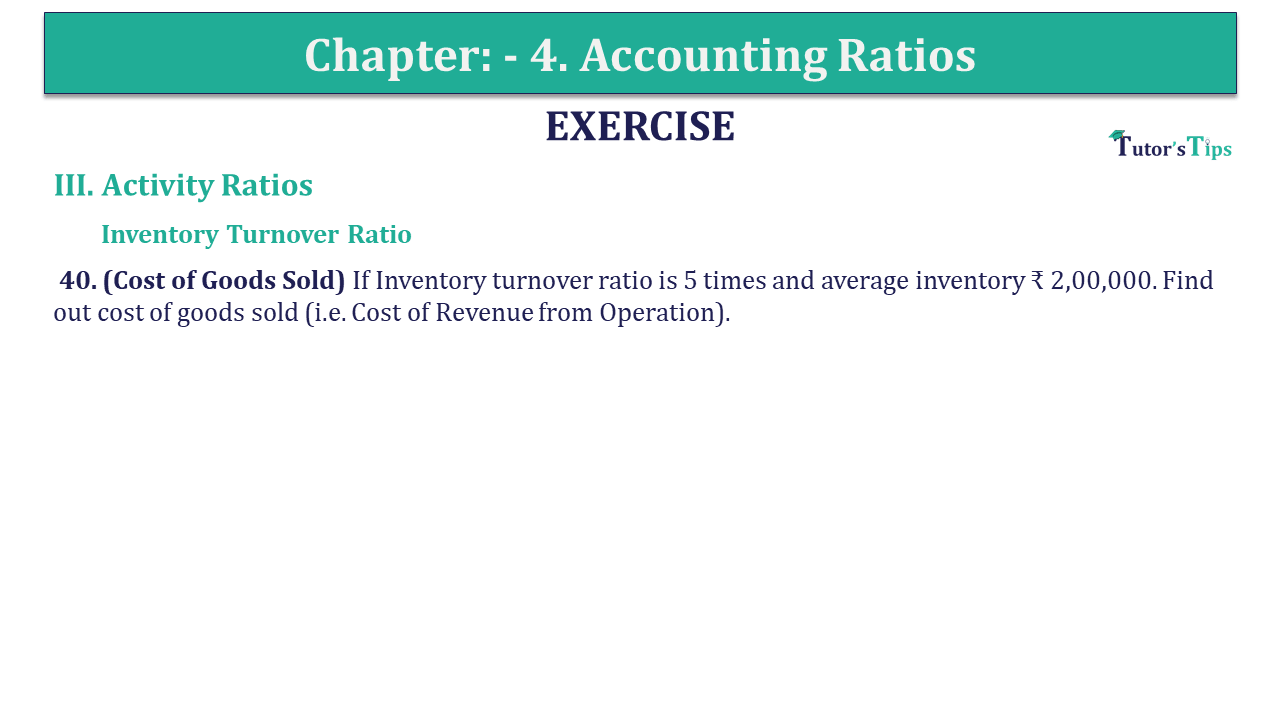# Question 40 Chapter 4 of +2-B – USHA Publication 12 ClassQ-40- CH-4 Book 2 - Usha Pub. +2 Book 2020 - Solution

Question 40 Chapter 4 of +2-B

III. Activity Ratios

Inventory Turnover Ratio

40. (Cost of Goods Sold) If the Inventory turnover ratio is 5 times and the average inventory ₹ 2,00,000. Find out the cost of goods sold (i.e. Cost of Revenue from Operation).

## The solution of Question 40 Chapter 4 of +2-B: –

 Inventory Turnover Ratio = 5 Times Average Inventory = ₹ 2,00,000
 Inventory Turnover Ratio = Cost of goods sold Average Inventory
 5 = Cost of goods sold ₹ 2,00,000 Cost of goods sold = ₹ 2,00,000 x 5 = ₹ 10,00,000

Also, Check out the solved question of previous Chapters: –